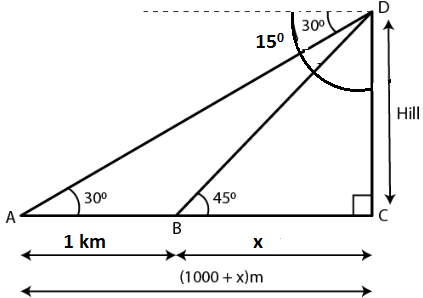Guru

# From the top of a hill, the angles of depression of two consecutive kilometer stones, due east are found to be 300 and 450 respectively. Find the distance of two stones from the foot of the hill.

• 0

sir this is the important  question from the book -ML aggarwal( avichal publication) class 10th , chapter20 , heights and distances
we have that From the top of a hill,
the angles of depression of two consecutive kilometer stones,
due east are found to be 300 and 450 respectively.
now we have Find the distance of two stones from the foot of the hill.

question no 19 , heights and distances , ICSE board

Share

1. Consider A and B as the position of two consecutive kilometre stones

Here AB = 1 km = 1000 m

Take Distance BC = x m

Distance AC = (1000 + x) mIn right angled triangle BCD

CD/BC = tan 450

So we get

CD/BC = 1

CD = BC = x

In right angled triangle ACD

DC/AC = tan 300

x/ (x + 1000) = 1/√3

By cross multiplication

√3x = x + 1000

(√3 – 1)x = 1000

x = 1000/ (√3 – 1)

We can write it as

x = [1000/(√3 – 1) × (√3 + 1)/ (√3 + 1)]

x = [1000 (√3 + 1)/ (3 – 1)]

x = [1000 (√3 + 1)/ 2]

x = 500 (1.73 + 1)

So we get

x = 500 × 2.73

x = 1365 m

Here the distance of first stone from the foot of hill = 1365 m

Distance of the second stone from the foot of hill = 1000 + 1365 = 2365 m

• -1